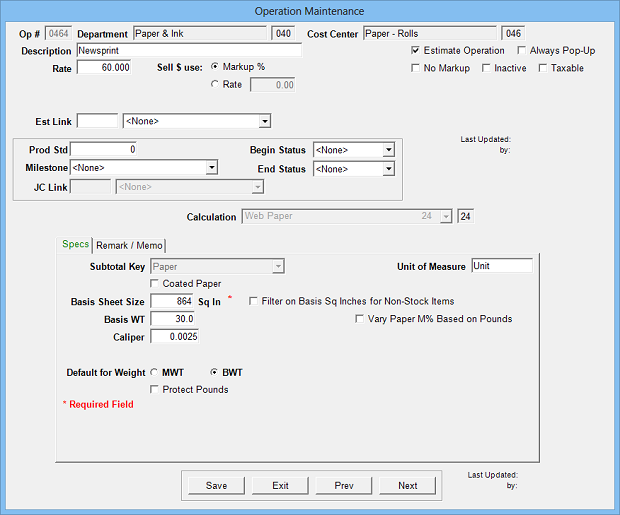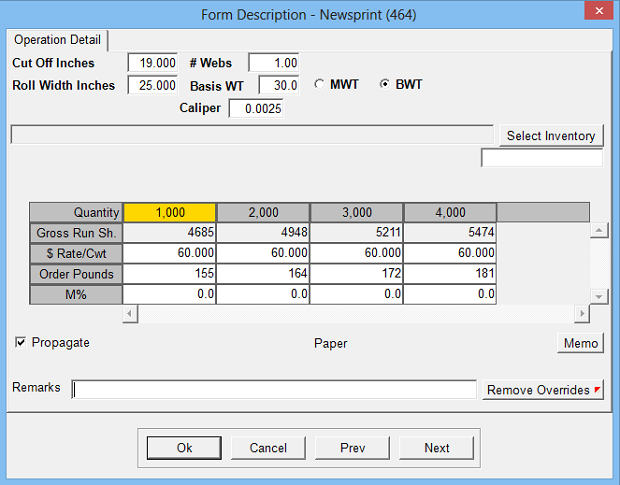Estimates pounds and cost of web paper based on web press run. Different weights of similar paper may be entered as one operation.

Operation Setup Requirements:
1. Description
2. Rate: Cost per 100 pounds of paper
3. Sell \$ use: Markup % (recommended) or alternate sell rate
4. Calc: 24
5. Sub Key: Paper Type
6. Paper Type: Coated or Non-Coated (used only by Calc 12: Ink)
7. Basis Size Sheet: Square inches of basis sheet size
8. Basis WT: Option to define default basis weight for paper
9. Caliper: Paper Caliper (optional, used by cutting by caliper and max press speeds by caliper)
10. Filter on Basis Square Inches for Non-Stock: Makes available only Non-Stock items with matching Basis Square Inch definition
11. Default for Weight: Basis WT or MWT
12. Protect Pounds: Prevents override of calculated paper
13. Vary Paper M% Based on Pounds: A table will appear when checked to apply markup percentages based on total order poundsEstimate Specification Input Prompts:

1. Cut off in inches (defaults to run length)
2. Roll width inches (defaults to run width)
3. # Webs
4. Basis Weight (or MWT): Default weight of paper, modifiable
5. Caliper: Default caliper of paper, modifiable
6. \$Rate/CWT: Cost per 100 pounds of paper
7. Order Pounds: Weight of equivalent gross run sheets (plus makeready sheets if calc 6 ‘order MR sheets’ selected)
8. Markup %
9. Remarks
10. Memo
11. Select Inventory: Option to select specific inventory item (Available if Inventory active)Calculation:

((Equiv Gross Run Sheets / # Web Stands) + Equiv MR Sheets) x # Webs = Equiv Order Sheets

Order Pounds (if MWT) = (Equiv Order Sheets x MWT)/1000

Order Pounds (if BWT) = (Equiv Order Sheets x Order Size x BWT) / (500 x Basis Size)

Paper Cost \$ = (Order Pounds / 100) x \$ Rate

Paper Cost \$ x Markup % = Markup \$

Paper Cost \$ + Markup \$ = Sell \$